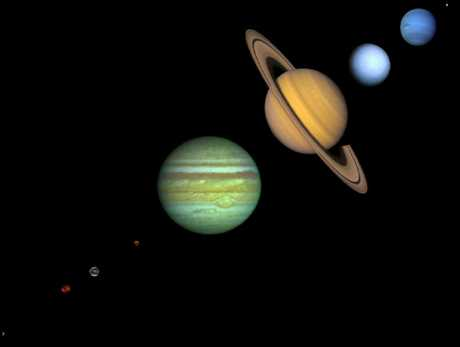Newton's Three Laws
of Motion

Let us begin our explanation of how Newton changed our understanding of the Universe by enumerating his Three Laws of Motion.

### Newton's First Law of Motion:

 I. Every object in a state of uniform motion tends to remain in that state of motion unless an external force is applied to it.

This we recognize as essentially Galileo's concept of inertia, and this is often termed simply the "Law of Inertia".

### Newton's Second Law of Motion:

 II. The relationship between an object's mass m, its acceleration a, and the applied force F is F = ma. Acceleration and force are vectors (as indicated by their symbols being displayed in slant bold font); in this law the direction of the force vector is the same as the direction of the acceleration vector.

This is the most powerful of Newton's three Laws, because it allows quantitative calculations of dynamics: how do velocities change when forces are applied. Notice the fundamental difference between Newton's 2nd Law and the dynamics of Aristotle: according to Newton, a force causes only a change in velocity (an acceleration); it does not maintain the velocity as Aristotle held.

This is sometimes summarized by saying that under Newton, F = ma, but under Aristotle F = mv, where v is the velocity. Thus, according to Aristotle there is only a velocity if there is a force, but according to Newton an object with a certain velocity maintains that velocity unless a force acts on it to cause an acceleration (that is, a change in the velocity). As we have noted earlier in conjunction with the discussion of Galileo, Aristotle's view seems to be more in accord with common sense, but that is because of a failure to appreciate the role played by frictional forces. Once account is taken of all forces acting in a given situation it is the dynamics of Galileo and Newton, not of Aristotle, that are found to be in accord with the observations.

### Newton's Third Law of Motion:

 III. For every action there is an equal and opposite reaction.

This law is exemplified by what happens if we step off a boat onto the bank of a lake: as we move in the direction of the shore, the boat tends to move in the opposite direction (leaving us facedown in the water, if we aren't careful!).